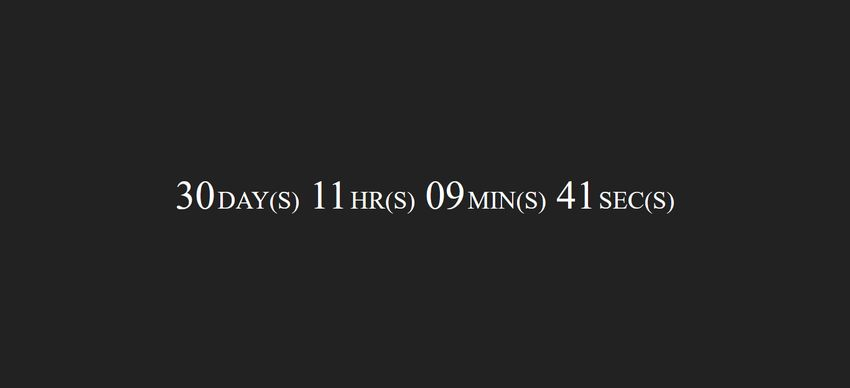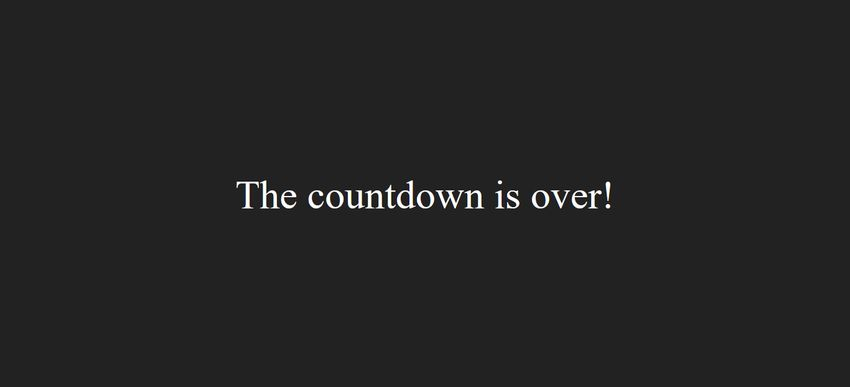# How to Build a Countdown Timer in Pure JavaScript

You don’t necessarily need jQuery to add a countdown timer to your site. It takes just a few lines of JavaScript to create a customizable timer that counts down to a pre-defined date and time. You can embed the timer to several kinds of pages such as Coming Soon and Maintenance pages, eCommerce stores, daily deals websites, and event registration pages.

In this tutorial, we will create the countdown timer step by step so that you can understand everything. You can find the entire JavaScript code at the end of the article.

### 1. Create the HTML

Let’s start with the HTML. The countdown timer will display four figures: days, hours, minutes, and seconds. Create a <span> element for each so that later you can target them with JavaScript.

Also add a <script> tag with the path to the JavaScript file right before the closing <body> tag.

```<div class="container">
<p id="timer">
<span id="timer-days"></span>
<span id="timer-hours"></span>
<span id="timer-mins"></span>
<span id="timer-secs"></span>
</p>
</div>
<script src="script.js"></script>```

### 2. Set an End Date

Create a new global variable for the date and time when the countdown will expire (endDate in the example). You can define its value by creating a new Date object and calling its getTime() method.

The endDate variable will hold the expiry date in UTC format that shows the milliseconds since Jan 1, 1970, 00:00:00.000 GMT. For example, the UTC value of Mar 15, 2019, 12:00:00 is 1552647600000.

`var endDate = new Date("Mar 15, 2019 12:00:00").getTime();`

### 3. Define the Timer

Create another global variable for the timer. The example below uses a function expression, as this way you don’t have to call the function separately. However, if you want you can also declare it as a function statement.

The setInterval() global JavaScript method repeatedly executes a function. Its first argument is the function to be executed and the second argument is the delay in-between executions. You need to declare the delay in milliseconds. Here, it will be 1000, as you want to update the timer every second (1 second = 1000 milliseconds).

```var timer = setInterval(function() {

/* Here comes the rest of JavaScript code. */

}, 1000);```

### 4. Calculate the Remaining Time

Inside the timer() function, define two new local variables. The first one (now) will return the current time and the other one (t) will calculate the remaining time.

In JavaScript, an empty Date() object returns the current date and time. The now variable calls its getTime() method and stores the current time in UTC format. The t variable calculates the remaining time by deducting the current time from the end date.

```let now = new Date().getTime();
let t = endDate - now;
```

### 5. Convert UTC Time to Days, Hours, Minutes & Seconds

Create an if block that will hold the instructions belonging to the timer. This code will be executed every second until the remaining time is greater than zero. By using an if block, you don’t have to stop the timer when the countdown is over.

First, you need to convert the remaining time into a usable format. Currently, it’s in UTC format, while you need to display it as days, hours, minutes, and seconds. So, declare four new local variables for the remaining days, hours, minutes, and seconds.

```if (t >= 0) {

let days = Math.floor(t / (1000 * 60 * 60 * 24));
let hours = Math.floor((t % (1000 * 60 * 60 * 24)) / (1000 * 60 * 60));
let mins = Math.floor((t % (1000 * 60 * 60)) / (1000 * 60));
let secs = Math.floor((t % (1000 * 60)) / 1000);

}```

Using the Math.floor() built-in JavaScript function, you can round down any float value to the nearest integer. The rest of the calculations are as follows:

• Remaining days: Divide the UTC value by 1000 * 60 * 60 * 24 which is the number of milliseconds in a single day (milliseconds * seconds * minutes * hours).
• Remaining hours: Get the remainder of the previous calculation using the % remainder operator and divide it by the number of milliseconds in a single hour (1000 * 60 * 60 = milliseconds * seconds * minutes).
• Remaining minutes: Get the remaining minutes and divide it by the number of milliseconds in a single minute (1000 * 60 = milliseconds * seconds).
• Remaining seconds: Get the remaining seconds and divide it by the number of milliseconds in a single second (1000).

### 6. Output the Timer

Now that you have all the data in the right format, you can output the timer to the screen. The getElementById() method of the document object allows you to target the HTML elements you created in Step 1, respectively #timer-days, #timer-hours, #timer-mins, and #timer-secs. You can place content into the targeted HTML elements with the innerHTML property.

Add the following code to the if (t >= 0) block, below the time conversion calculations:

```document.getElementById("timer-days").innerHTML = days +
"<span class='label'>DAY(S)</span>";

document.getElementById("timer-hours").innerHTML= ("0" + hours).slice(-2) +
"<span class='label'>HR(S)</span>";

document.getElementById("timer-mins").innerHTML= ("0" + mins).slice(-2) +
"<span class='label'>MIN(S)</span>";

document.getElementById("timer-secs").innerHTML= ("0" + secs).slice(-2) +
"<span class='label'>SEC(S)</span>";```

The code above adds a “0” character to hours, minutes, and seconds when their values are less than 10. Although this is not necessary, the timer looks better when the number of digits doesn’t change all the time on the screen.

This can be achieved with a formatting trick that makes use of the slice() method that can be used to return a portion of a data set. For instance, slice(1) slices off the first character of a string and returns the rest of the string. Similarly, slice(2) removes the first two characters and returns the rest. A negative value returns the targeted characters rather than slicing them off. So, slice(-2) returns the last two characters of a string.

In the code, you need to prepend every digit with a “0” and return the last two characters with slice(-2). This way JavaScript will add a leading zero to every one-digit number but leave two-digit numbers intact. For example:

• (“05”).slice(-2) = “05”;
• (“018”).slice(-2) = “18”;

### 7. Notify Users When the Countdown Is Over

You can notify users when the countdown is over if you want. Create an else block and target the #timer element with the getElementById() method. You can add any text as innerHTML you want to display to users when the countdown expires.

```else {

document.getElementById("timer").innerHTML = "The countdown is over!";

}```

Alternatively, you can omit the entire else block, in which case the timer will simply disappear from the screen when the countdown is over.

### 8. Put It Together

And, that’s all! Here is the full JavaScript code you need to use to create the countdown timer:

```var endDate = new Date("Mar 15, 2019 12:00:00").getTime();

var timer = setInterval(function() {

let now = newDate().getTime();
let t = endDate - now;

if (t >= 0) {

let days = Math.floor(t / (1000 * 60 * 60 * 24));
let hours = Math.floor((t % (1000 * 60 * 60 * 24)) / (1000 * 60 * 60));
let mins = Math.floor((t % (1000 * 60 * 60)) / (1000 * 60));
let secs = Math.floor((t % (1000 * 60)) / 1000);

document.getElementById("timer-days").innerHTML = days +
"<span class='label'>DAY(S)</span>";

document.getElementById("timer-hours").innerHTML = ("0"+hours).slice(-2) +
"<span class='label'>HR(S)</span>";

document.getElementById("timer-mins").innerHTML = ("0"+mins).slice(-2) +
"<span class='label'>MIN(S)</span>";

document.getElementById("timer-secs").innerHTML = ("0"+secs).slice(-2) +
"<span class='label'>SEC(S)</span>";

} else {

document.getElementById("timer").innerHTML = "The countdown is over!";

}

}, 1000);```

You can style the countdown timer any way you want. The CSS below uses flexbox to position it to the center of the screen both horizontally (with justify-content) and vertically (with align-items).

```body {
margin: 0;
}
.container {
background: #222;
width: 100%;
height: 100vh;
display: flex;
justify-content: center;
align-items: center;
}
#timer {
color: #fff;
font-size: 4rem;
}
.label {
font-size: 2.5rem;
}```

### Final Result

Here is how the countdown timer using the above CSS looks like. When the timer is running it shows the days, hours, minutes, and seconds:When the countdown is over, it displays a notification to users:### Next Steps

You can build many cool things with JavaScript—sometimes with just a few lines of code.

If you want to learn more, have a look at our article about the four techniques you can use to create a JavaScript object.

We also have an excellent collection of 11 experimental JavaScript projects that show how far the boundaries of JavaScript can be pushed (beware, you might be surprised!).